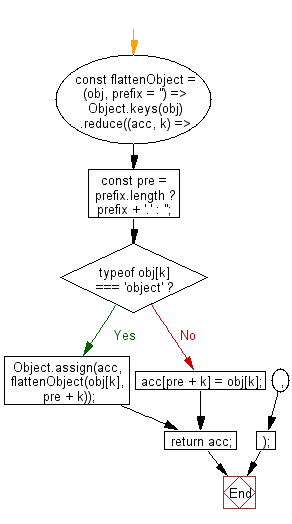# JavaScript: Flatten an object with the paths for keys

## JavaScript fundamental (ES6 Syntax): Exercise-233 with Solution

Write a JavaScript program to flatten an object with the paths for keys.

• Use recursion.
• Use Object.keys(obj) combined with Array.prototype.reduce() to convert every leaf node to a flattened path node.
• If the value of a key is an object, the function calls itself with the appropriate prefix to create the path using Object.assign().
• Otherwise, it adds the appropriate prefixed key-value pair to the accumulator object.
• You should always omit the second argument, prefix, unless you want every key to have a prefix.

Sample Solution:

JavaScript Code:

``````//#Source https://bit.ly/2neWfJ2
const flattenObject = (obj, prefix = '') =>
Object.keys(obj).reduce((acc, k) => {
const pre = prefix.length ? prefix + '.' : '';
if (typeof obj[k] === 'object') Object.assign(acc, flattenObject(obj[k], pre + k));
else acc[pre + k] = obj[k];
return acc;
}, {});
console.log(flattenObject({ a: { b: { c: 1 } }, d: 1 }));
```
```

Sample Output:

```{"a.b.c":1,"d":1}
```

Flowchart:Live Demo:

See the Pen javascript-basic-exercise-233-1 by w3resource (@w3resource) on CodePen.

Improve this sample solution and post your code through Disqus

What is the difficulty level of this exercise?

Test your Programming skills with w3resource's quiz.

﻿